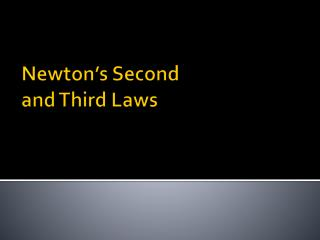Download PresentationNewton’s Second and Third Laws

# Newton’s Second and Third Laws - PowerPoint PPT Presentation

Newton’s Second and Third Laws. Acceleration and Force. Acceleration is directly Proportional to the Force Acceleration α Net Force If the net force is increased, then the acceleration must increase by the same factor as long as mass is held constant. Force and Acceleration.I am the owner, or an agent authorized to act on behalf of the owner, of the copyrighted work described.
Download Presentation## Newton’s Second and Third Laws

An Image/Link below is provided (as is) to download presentation

Download Policy: Content on the Website is provided to you AS IS for your information and personal use and may not be sold / licensed / shared on other websites without getting consent from its author.While downloading, if for some reason you are not able to download a presentation, the publisher may have deleted the file from their server.

- - - - - - - - - - - - - - - - - - - - - - - - - - E N D - - - - - - - - - - - - - - - - - - - - - - - - - -
Presentation Transcript
1. Newton’s Second and Third Laws

2. Acceleration and Force • Acceleration is directly Proportional to the Force • Acceleration α Net Force • If the net force is increased, then the acceleration must increase by the same factor as long as mass is held constant.

3. Force and Acceleration • Acceleration is always in the direction of the net force.

4. Acceleration and Mass • Acceleration is inversely proportional to the mass of the object. • Accelerationα • If the mass increases, then the acceleration decreases as long as the force remains constant. • If the mass is doubled, then the acceleration is cut in half.

5. Force, Mass and Acceleration • The acceleration is directly proportional to the net force divided by the mass • Accelerationα

6. Newton’s 2nd Law of Motion • Newton’s Second Law – The acceleration of an object is directly proportional to the net external force acting on the object and is inversely proportional to the mass of the object. • Fnet= ma

7. Equation Variables and Units • Newton’s Second Law variables • Fnet: net force (Newton – N) • m: mass (Kilograms – kg) • a: acceleration (meters per second² - m/s²)

8. Example Problem #1 • What net force is needed to move a 3.2kg book across a table with an acceleration of 2.1 m/s² to the right? • Answer: 6.7 N to the right

9. Example Problems #2 • The net force on the propeller of a 0.75kg model airplane is 17 N forward. What is the acceleration of the airplane?

10. Example Problem #3 • A ball pushed with a net force of 13.5 N to the right accelerates at 6.5 m/s² to the right. What is the mass of the ball?

11. Example Problem #4 • Two people push on a box resting on a frictionless floor. One person pushes to the left with a force of 17 N and the other person pushed with a force of 37 N to the right. If the mass of the box is 10.0 kg, what is the acceleration of the box?

12. Newton’s 3rd Law • Newton’s Third Law – If two bodies interact, the magnitude of the force exerted on object 1 by object 2 is equal to the magnitude of the force simultaneously exerted on object 2 by object 1, and these two forces are opposite in direction. • For every action, there is an equal and opposite reaction.

13. Forces Always Exist in Pairs • Forces always exist in pairs. • If you push on a wall with 100 N, the wall presses back on you with 100 N. • Equal and opposite, as long as there is no acceleration. • If Earth is pulling you down with a force equal to your weight, what is the second force?

14. Action-Reaction Pair • Action-Reaction Pair – A pair of simultaneous equal but opposite forces resulting from the interaction of two objects. • The action and reaction occur at the same exact time.

15. Field Forces • Field Forces also exist in pairs as well. • Gravity is an example of a field force. • If you drop a ball the Earth pulls down on the ball, but the ball pulls up on the Earth by the same amount. • But why doesn’t the earth move and the ball does?